# 论文引入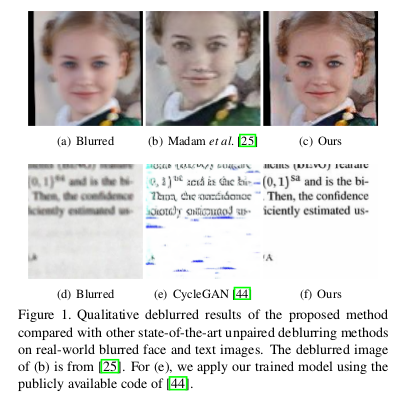• 内容编码器和模糊编码器将模糊图像的内容和模糊特征区分开，实现了高质量的图像去模糊。
• 对模糊编码器添加KL散度损失以阻止模糊特征对内容信息进行编码。
• 为了保留原始图像的内容结构，在框架中添加了模糊图像构造和循环一致性损失，同时添加的感知损失有助于模糊图像去除不切实际的伪像。

# 模型详解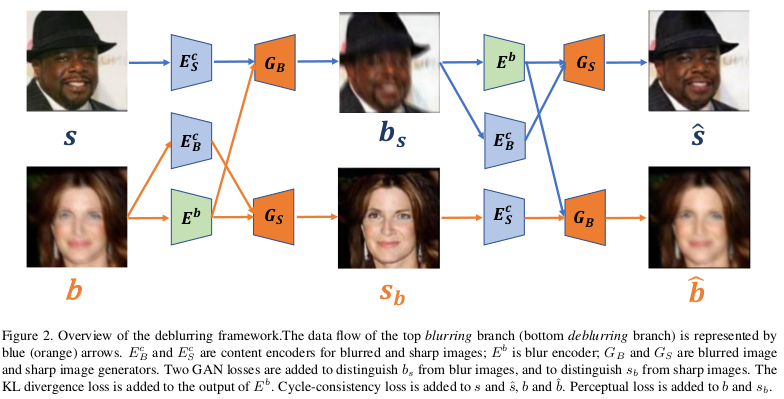# 模型损失函数

$KL(q(z_b)\Vert p(z)) = - \int q(z_b)log \frac{p(z)}{q(z_b)}dz$

$\mathcal L_{KL} = \frac{1}{2} \sum_{i=1}^N(\mu_i^2 + \sigma_i^2 - log(\sigma_i^2) -1)$

$\mathcal L_{D_s} = \mathbb E_{s \sim p(s)}[logD_s(s)] + \mathbb E_{b \sim p(b)}[log(1-D_s(G_s(E_B^c(b),z_b)))]$

$\mathcal L_{cc} = \mathbb E_{s \sim p(s)}[\Vert s - \hat{s} \Vert_1] + \mathbb E_{b \sim p(b)}[\Vert b - \hat{b} \Vert_1]$

$\mathcal L_{p} = \Vert \phi_l(s_b) - \phi_l(b) \Vert_2^2$

$\mathcal L = \lambda_{adv} \mathcal L_{adv} + \lambda_{KL} \mathcal L_{KL} + \lambda_{cc} \mathcal L_{cc} + \lambda_{p} \mathcal L_{p}$

# 实验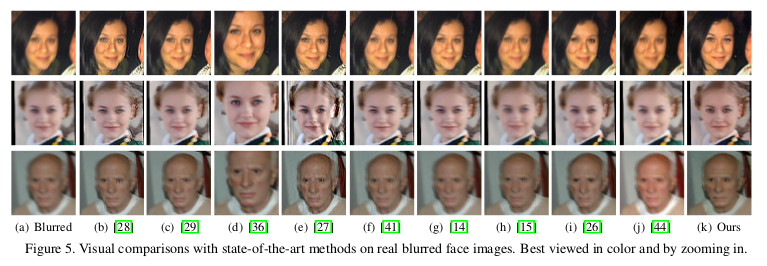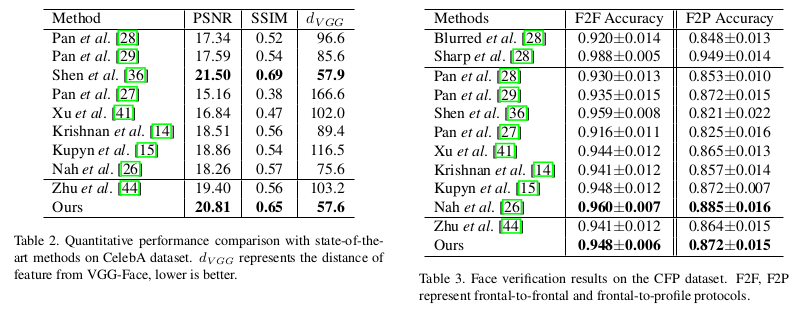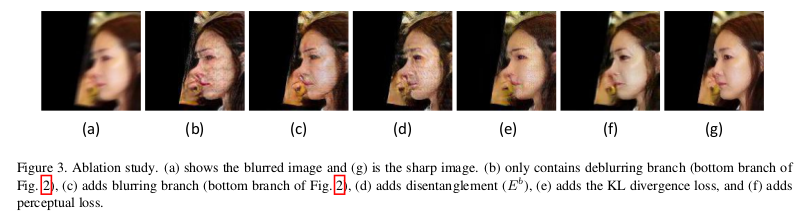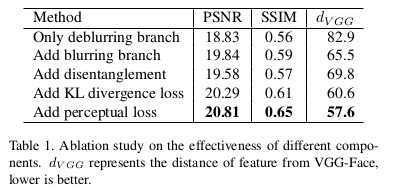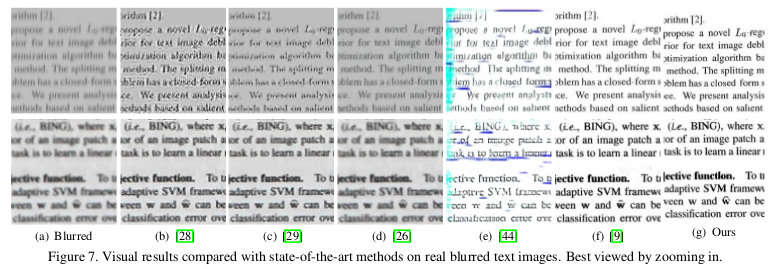# 参考文献

 T. Madam Nimisha, K. Sunil, and A. Rajagopalan. Unsupervised class-specific deblurring. In Proceedings of the European Conference on Computer Vision (ECCV), pages 353–369, 2018.

 J.-Y. Zhu, T. Park, P. Isola, and A. A. Efros. Unpaired image-to-image translation using cycle-consistent adversarial networks. In Proceedings of International Conference on Computer Vision (ICCV), 2017.

 H.-Y. Lee, H.-Y. Tseng, J.-B. Huang, M. Singh, and M.-H.Yang. Diverse image-to-image translation via disentangled representations. In Proceedings of European Conference on Computer Vision (ECCV), pages 36–52. Springer, 2018.